# Verbal Reasoning - Character Puzzles

### Exercise :: Character Puzzles - Character Puzzles 1

46.

Which one will replace the question mark ?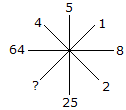A. 1 B. 2 C. 3 D. 4

Explanation:

(2)2 = 4

(8)2 = 64

(5)2 = 25

(1)2 = 1.

47.

Which one will replace the question mark ?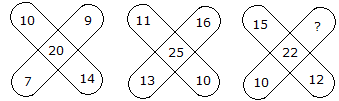A. 6 B. 7 C. 8 D. 9

Explanation:

(10 + 9 + 14 + 7)/2 = 20

and (11 + 16 + 10 + 13)/2 = 25

Therefore, (15 + ? + 12 + 10)/2 = 22

Hence 37 + ? = 44

? = 44 - 37

? = 7.

48.

Which one will replace the question mark ?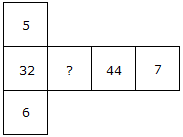A. 33 B. 38 C. 32 D. 37

Explanation:

(5 x 6) + 2 = 32

(7 x 6) + 2 = 44

(7 x 5) + 2 = 37.

49.

Which one will replace the question mark ?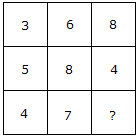A. 6 B. 7 C. 8 D. 9

Explanation:

(5 + 3)/2 = 4

and (6 + 8)/2 = 7

Therefore (8 + 4)/2 = 6.

50.

Which one will replace the question mark ?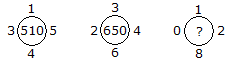A. 660 B. 670 C. 610 D. 690

Explanation:

(1)2 + (5)2 + (4)2 + (3)2 = 51 x 10 = 510

and (3)2 + (4)2 + (6)2 + (2)2 = 65 x 10 = 650

Similarly (0)2 + (1)2 + (2)2 + (8)2 = 69 x 10 = 690.